# Creating KPI views: the steps + the cheat sheet with formulas

Modified on Wed, 01 Jun 2022 at 06:18 PM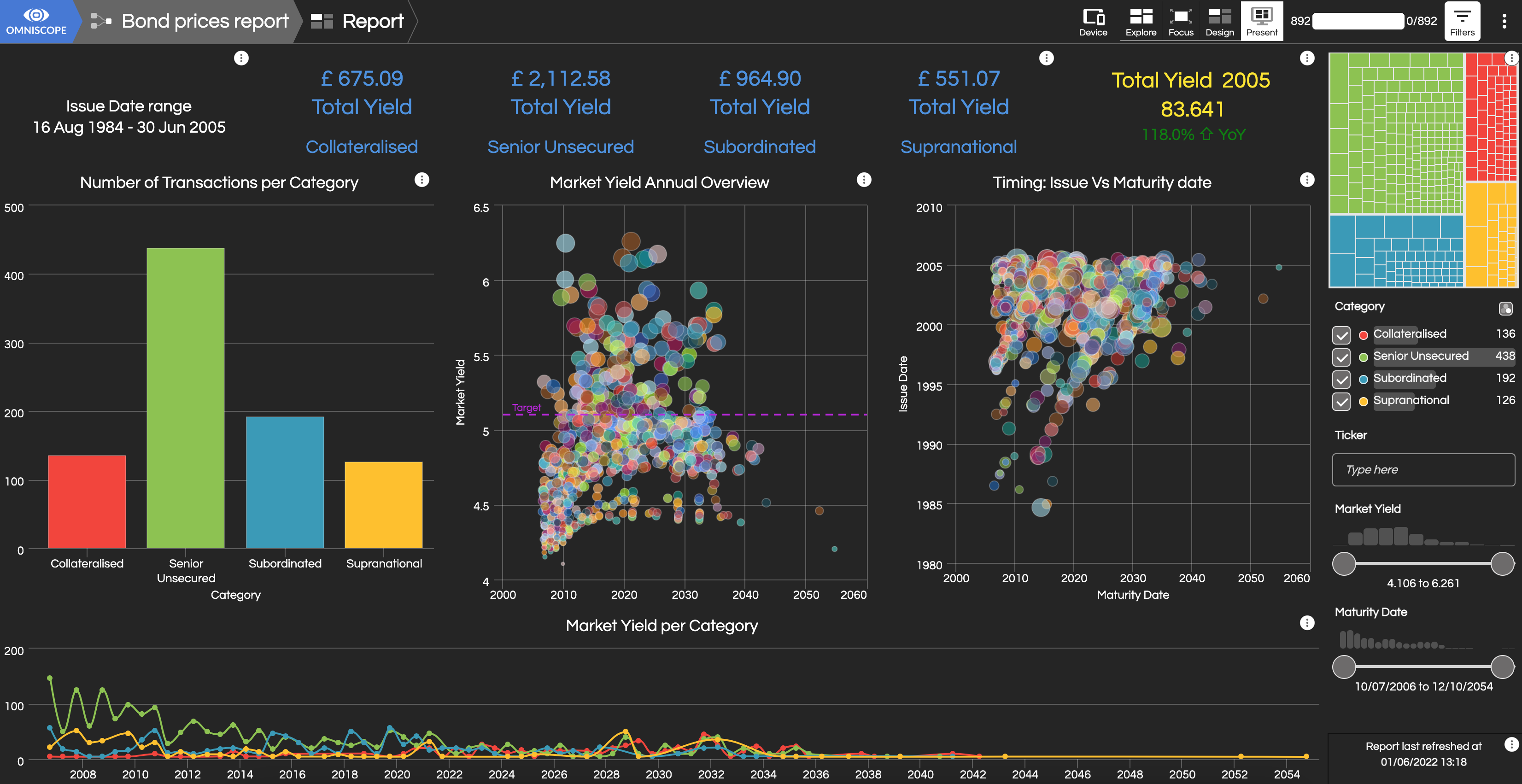Creating a dynamic self-updating dashboard is a time-saver for the analysts - it also means that the time it takes to get from a data refresh to a polished visualisation is reduced to a couple of seconds.

KPI views are a great addition to charts - KPIs will highlight the totals and show periodic changes - sometimes all the decision-makers need in order to comprehend the latest business performance.

There is also an article on this portal explaining use of calculations in different areas of Omniscope application, however here we'll cover just some KPI examples that you can immediately implement in your reports.

## How to create a KPI view - steps 1-2-3The KPI calculation in this view will depend on the Data and Query choices you select from the Settings menu.

2. Pick the dataset from the Data menu: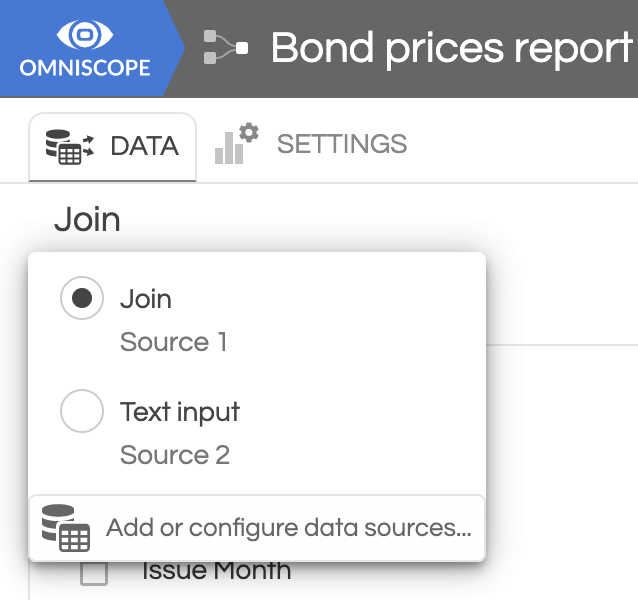3. From the Settings > Query options pick one of the options:

- 'Filtered in' - default setting, where calculation is based on the filtered data, so anything filtered out will be ignored

- 'Selection' - the result will be based on the data selected in another view (e.g. bar, marker, pie slice in a chart based on the same dataset!)

- 'All data' - your calculation will be performed on the whole dataset and will not respond to filtering on the tab. Simply delete the 'Filtered in' default by clicking on the bin button next to it.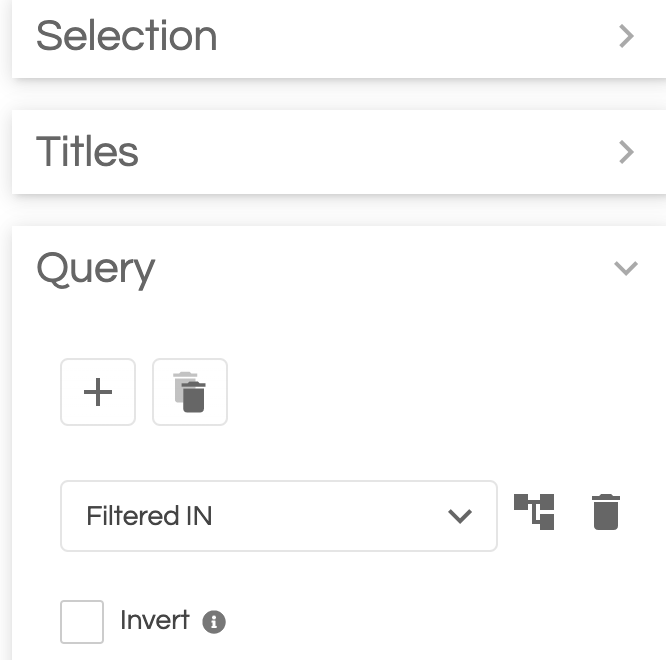4. Create some dynamic content!

Use the simple text editor to create and format a title and add your dynamic text by integrating formulas.

If it's a simple function (like sum, mean, min, max, etc.), based on one field - you can use the drop-down menu to pick the field name and function (below [Issue Date] with minimum).

There is also a formula editor interface (under the Custom formula), with the whole range of functions, allowing you to perform more sophisticated calculations in this space.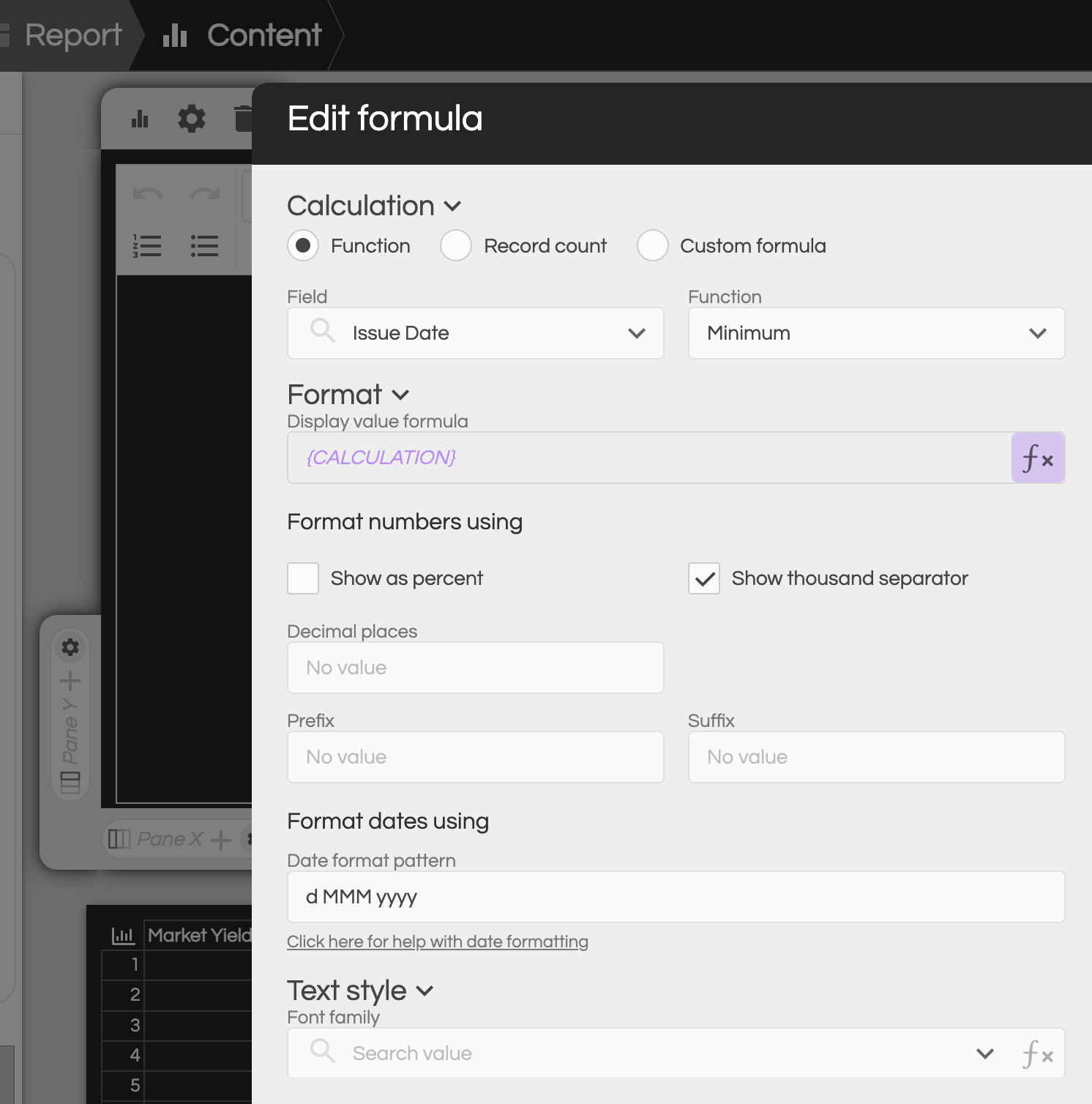By clicking on the 'Custom formula' you will open the Formula builder interface, where you can use the drop-down menus to add formula syntax and insert fields / variables from your dataset.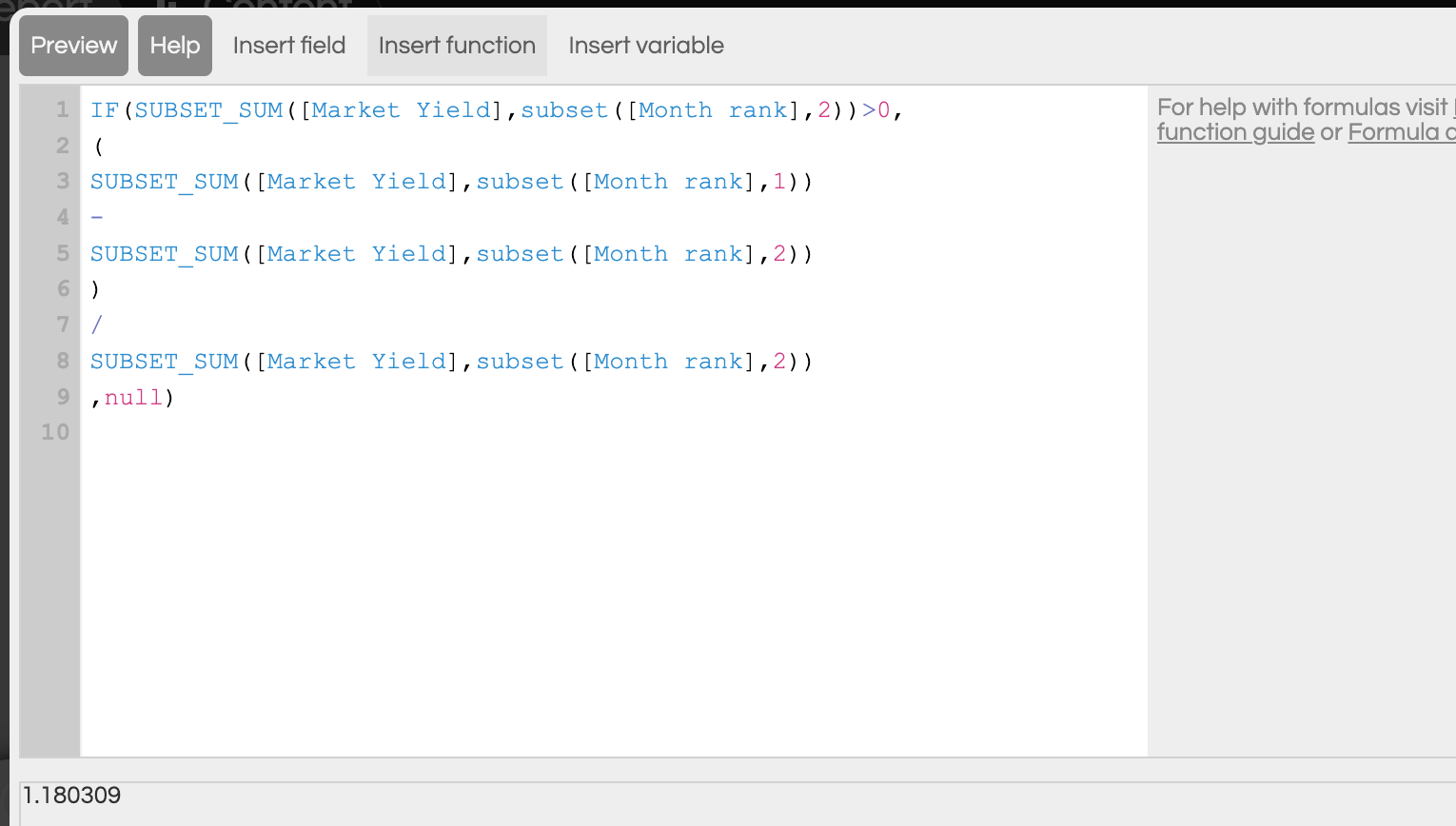In the Omniscope's Content view everything can be formula driven: including the text size, font choice, text colour, arrows and more. This allows the user to create KPIs numbers with different style, depending on the performance, so red colour/ italic font to draw attention to a bad performance, or use green font for good results.

Static text and formulas can be combined to create dynamic statements, that will change depending on the latest results e.g. "This week's sales were £250,865, which is 4% higher than last week(with a formula behind the numbers and the word "higher")

## The 'cheat sheet'

Here are some examples of the formulas that you may wish to adapt to your scenario. Please

note that because of the absence of a tabular data structure, referencing is done by using Subset functions with specific conditions, so Omniscope can determine on which subset of data to perform some statistical function.

#Sum of values on the last day in our time series:

SUBSET_SUM([Value], subset([Date],

SUBSET_MAX([Date])

))

#Previous day value:

SUBSET_SUM([Value], SUBSET([Date],

))

#Sum of Values on the same day last month (compared to the latest day in the series):

SUBSET_SUM([Value], subset([Date],

))

#the "month" argument can be replaced with "year","week" or "day". If Max date is 22 May 2022 - the formula will retrieve a sum of values for the 22nd April 2022, or, in case of -1 Year, sum of values on the 22 May 2021.

# finding the value sum on the last day of the previous month :

SUBSET_SUM([Value], subset([Date],

LASTDAYOFMONTH(

)))

#Value sum for the latest Month - our month field is called [Month MMM-yy]:

SUBSET_SUM([Value], subset([Month MMM-yy],

SUBSET_MAX([Month MMM-yy])

))

# MoM difference (month on month) from the latest month in our datset:

SUBSET_SUM([Value], subset([Month MMM-yy],

SUBSET_MAX([Month MMM-yy])

))

-

SUBSET_SUM([Value], subset([Month MMM-yy],

))

# Year to date Value sum (sum of all values for the latest year in the time series):

SUBSET_SUM([Value], subset([Year],

SUBSET_MAX([Year])

))

# Sum of values for the previous year:

SUBSET_SUM([Value], subset([Year],

))

# YoY (year on year) difference (sum of Values for the Max year - previous year):

SUBSET_SUM([Value], subset([Year],

SUBSET_MAX([Year])

))

-

SUBSET_SUM([Value], subset([Year],

))

#Difference from a previous day in a time series where [Value] field is a cumulative

SUBSET_MAX([Value])

-

SUBSET_MAX([Value], SUBSET([Date],

))

#Increase rate (delta divided by the previous period) -in a time series where [Value] field is a cumulative :

(SUBSET_MAX([Value])

-

SUBSET_MAX([Value], SUBSET([Date],

)))

/

SUBSET_MAX([Value], SUBSET([Date],

#Create a unicode arrow next to your result:

IF(

SUBSET_SUM([Market Yield],subset([Month rank],1))

-

SUBSET_SUM([Market Yield],subset([Month rank],2))

> 0, "⇧", "⇩")

# Colour the font or arrow depending on the calculation outcome (RGB colours can be used instead of words e.g. "rgb(255,0,0)" for red):

IF(

SUBSET_SUM([Market Yield],subset([Month rank],1))

-

SUBSET_SUM([Market Yield],subset([Month rank],2))

> 0,

"green", "red")

Below is a demo file which contains multiple conditional formulas - you can import the IOZ and explore the contents.